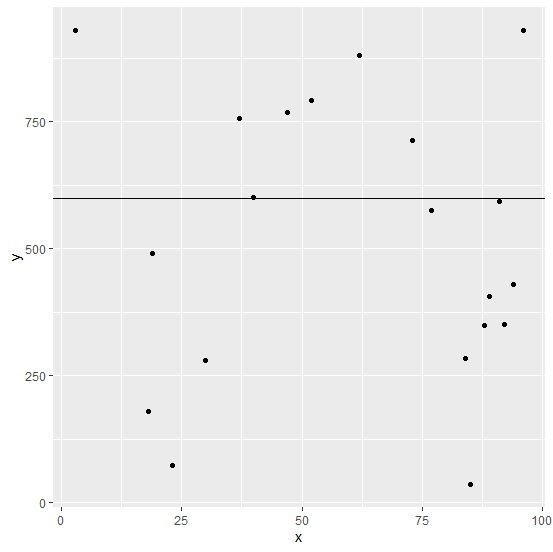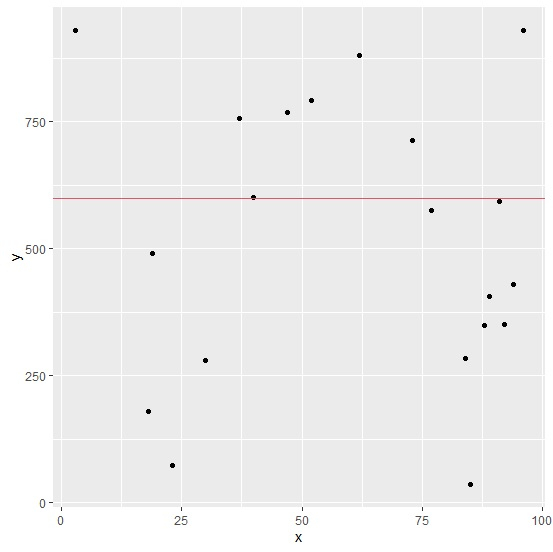# How to create a horizontal line in ggplot2 graph with different color in R?

To create a horizontal line in ggplot2 graph with different in R, we can follow the below steps −

• First of all, create a data frame.
• Then, create a plot using ggplot2 using geom_hline function having horizontal line.
• After that, create the same plot with line color defined with col argument.

## Create the data frame

Let's create a data frame as shown below −

Live Demo

x<-sample(1:100,20)
y<-sample(1:1000,20)
df<-data.frame(x,y)
df

On executing, the above script generates the below output(this output will vary on your system due to randomization) −

    x  y
1  85  35
2  91  593
3  19  491
4  40  601
5  84  283
6  18  179
7  88  349
8  47  769
9  30  279
10 62  881
11 3   930
12 94  429
13 77  576
14 52  792
15 37  757
16 89  405
17 73  713
18 23 72
19 96 931
20 92 350

## Create the plot with ggplot2

Using ggplot2 function to create the scatterplot between x and y with horizontal line at 600 −

x<-sample(1:100,20)
y<-sample(1:1000,20)
df<-data.frame(x,y)
library(ggplot2)
ggplot(df,aes(x,y))+geom_point()+geom_hline(yintercept=600)

### Output## Create the plot with colored horizontal line

Using col argument inside geom_hline to create the colored horizontal line −

x<-sample(1:100,20)
y<-sample(1:1000,20)
df<-data.frame(x,y)
library(ggplot2)
ggplot(df,aes(x,y))+geom_point()+geom_hline(yintercept=600,col=2)

### Output